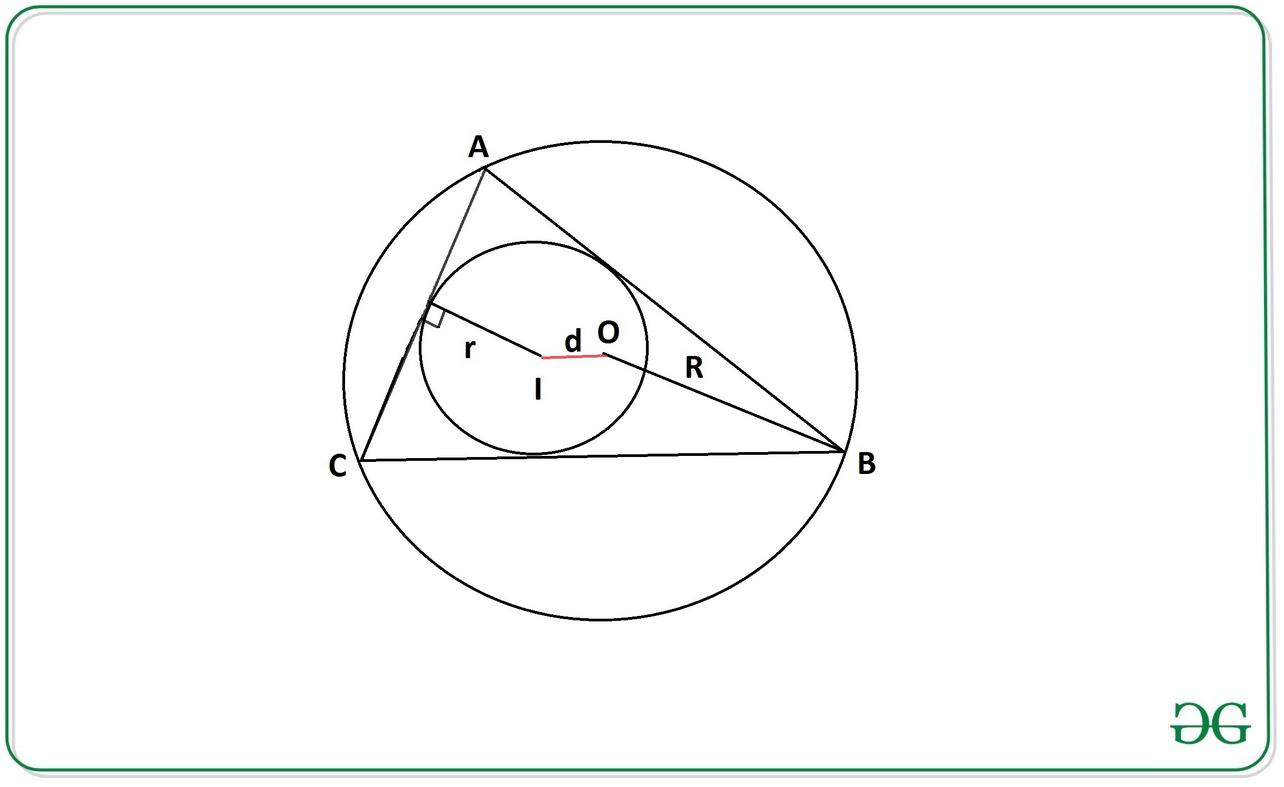# Distance between Incenter and Circumcenter of a triangle using Inradius and Circumradius

Given two integers r and R representing the length of Inradius and Circumradius respectively, the task is to calculate the distance d between Incenter and Circumcenter.Inradius The inradius( r ) of a regular triangle( ABC ) is the radius of the incircle (having center as l), which is the largest circle that will fit inside the triangle.
Circumradius: The circumradius( R ) of a triangle is the radius of the circumscribed circle (having center as O) of that triangle.

Examples:

Input: r = 2, R = 5
Output: 2.24

Input: r = 5, R = 12
Output: 4.9

Approach:
The problem can be solved using Euler’s Theorem in geometry, which states that the distance between the incentre and circumcentre of a triangle can be calculated by the equation:Below is the implementation of the above approach:

## Java

 // Java program for the above approach   import java.util.*;     class GFG{         // Function returns the required distance  static double distance(int r,int R)  {      double d = Math.sqrt(Math.pow(R, 2) -                           (2 * r * R));                                  return d;  }     // Driver code  public static void main(String[] args)  {             // Length of Inradius      int r = 2;             // Length of Circumradius      int R = 5;         System.out.println(Math.round(          distance(r, R) * 100.0) / 100.0);  }  }     // This code is contributed by offbeat

## Python3

 # Python3 program for the above approach  import math     # Function returns the required distance  def distance(r,R):         d = math.sqrt( (R**2) - (2 * r * R))             return d     # Driver Code     # Length of Inradius  r = 2    # Length of Circumradius  R = 5     print(round(distance(r,R),2))

## C#

 // C# program for the above approach   using System;     class GFG{         // Function returns the required distance  static double distance(int r, int R)  {      double d = Math.Sqrt(Math.Pow(R, 2) -                           (2 * r * R));                                 return d;  }     // Driver code  public static void Main(string[] args)  {             // Length of Inradius      int r = 2;             // Length of Circumradius      int R = 5;             Console.Write(Math.Round(          distance(r, R) * 100.0) / 100.0);  }  }     // This code is contributed by rutvik_56

Output:

2.24


Time Complexity: O(1)
Auxiliary Space: O(1)

Attention reader! Don’t stop learning now. Get hold of all the important DSA concepts with the DSA Self Paced Course at a student-friendly price and become industry ready.

My Personal Notes arrow_drop_upCheck out this Author's contributed articles.

If you like GeeksforGeeks and would like to contribute, you can also write an article using contribute.geeksforgeeks.org or mail your article to contribute@geeksforgeeks.org. See your article appearing on the GeeksforGeeks main page and help other Geeks.

Please Improve this article if you find anything incorrect by clicking on the "Improve Article" button below.

Improved By : offbeat, rutvik_56

Article Tags :
Practice Tags :

Be the First to upvote.

Please write to us at contribute@geeksforgeeks.org to report any issue with the above content.